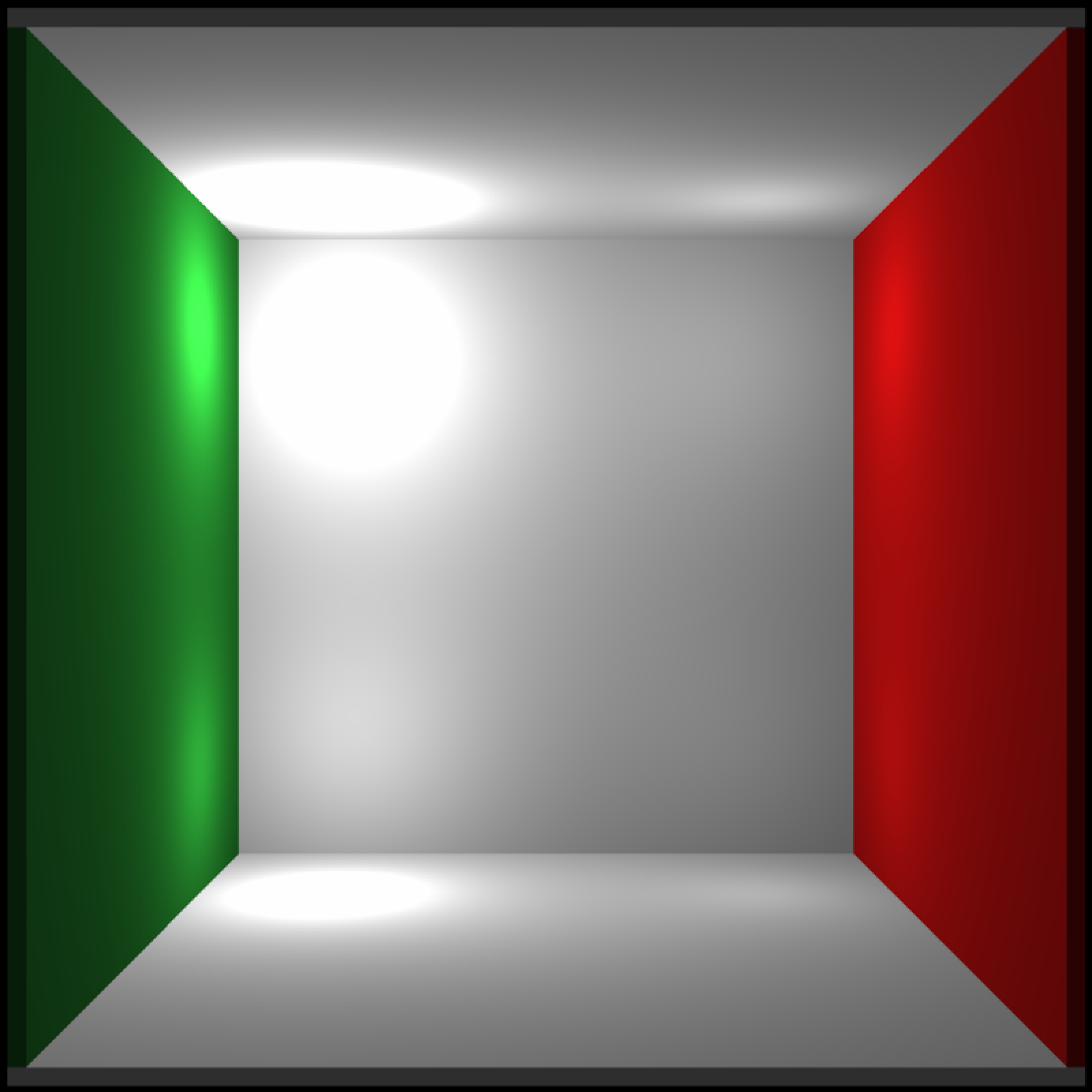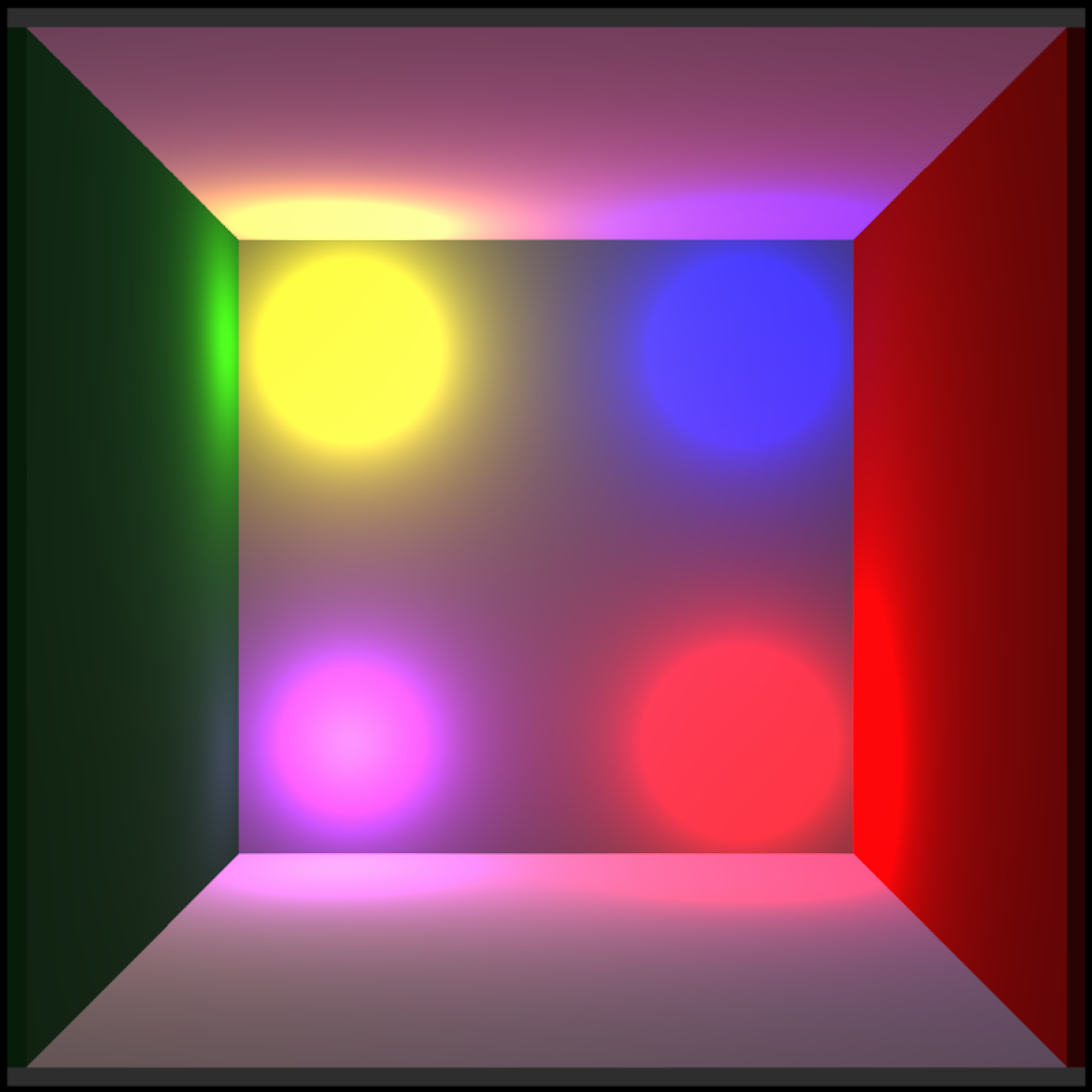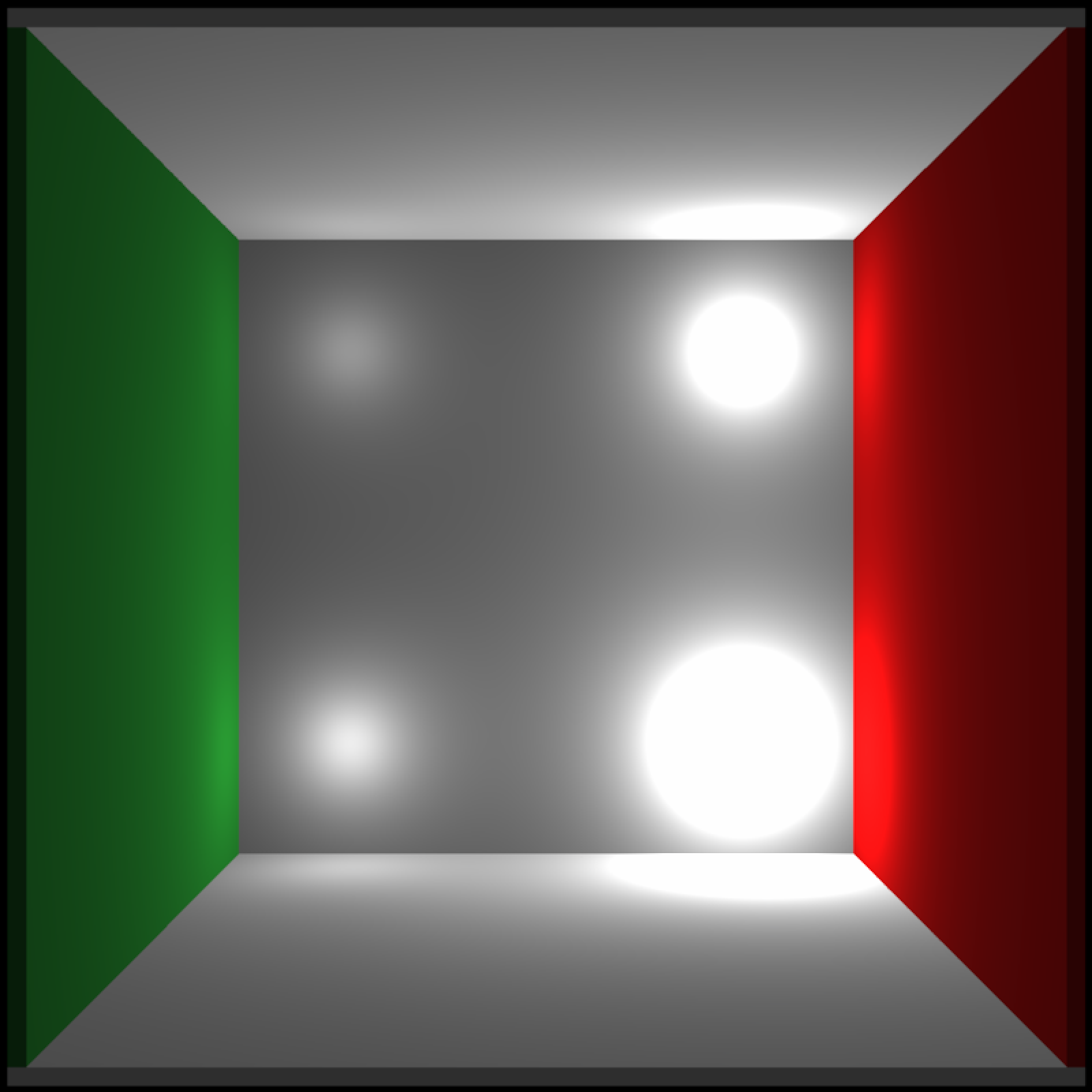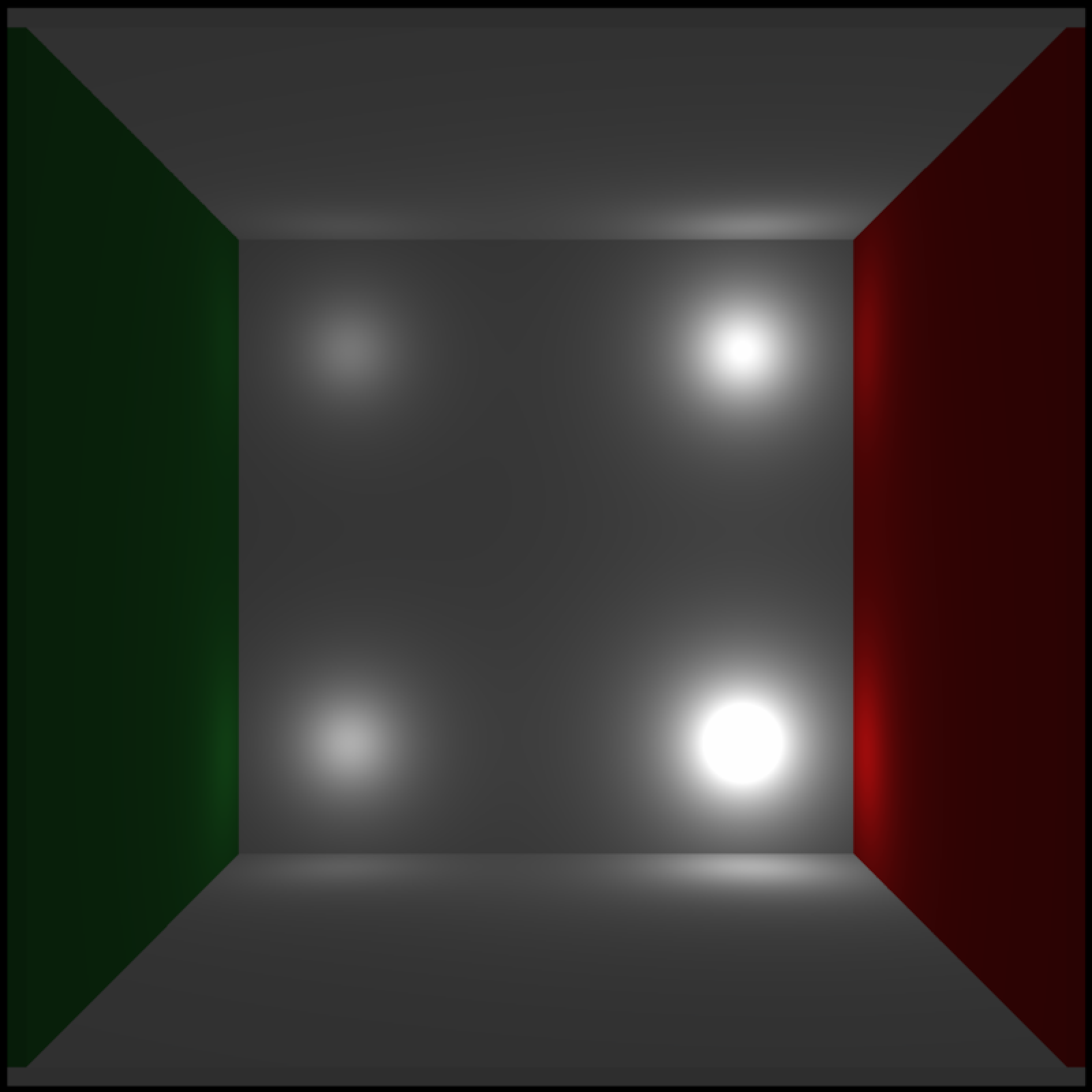The falloff of the point light intensity is given by the following equation (referenc:

Intensity = intensity / (constant + falloff * distance + falloff_quad * (distance * distance));

point_light(
position = c(0, 0, 0),
color = "white",
intensity = 1,
constant = 1,
falloff = 1,
)

## Arguments

position A two-dimensional matrix, where each entry in the matrix is the elevation at that point. All points are assumed to be evenly spaced. Default 400. Width of the rendered image. Default 1. Intensity of the point light. Default 1. Constant term. See description for details. Default 1. Linear falloff term. See description for details. Default 1. Quadratic falloff term. See description for details.

## Value

A matrix representing the light information.

## Examples

# \dontshow{
options("cores"=1)
# }
#Add point lights and vary the intensity
lights_int = point_light(position=c(100,100,400), color="white", intensity=0.125,
falloff_quad = 0.0, constant = 0.0002, falloff = 0.005) %>%
falloff_quad = 0.0, constant = 0.0002, falloff = 0.005)) %>%
falloff_quad = 0.0, constant = 0.0002, falloff = 0.005)) %>%
falloff_quad = 0.0, constant = 0.0002, falloff = 0.005))

# \donttest{
generate_cornell_mesh(light=FALSE) %>%
rasterize_scene(light_info = lights_int)
#> Setting default values for Cornell box: lookfrom c(278,278,-800) lookat c(278,278,0) fov 40 .# }
#Add point lights and vary the color
lights_c = point_light(position=c(100,100,500), color="red",
falloff_quad = 0.0, constant = 0.0002, falloff = 0.005) %>%
falloff_quad = 0.0, constant = 0.0002, falloff = 0.005)) %>%
falloff_quad = 0.0, constant = 0.0002, falloff = 0.005)) %>%
falloff_quad = 0.0, constant = 0.0002, falloff = 0.005))

# \donttest{
generate_cornell_mesh(light=FALSE) %>%
rasterize_scene(light_info = lights_c)
#> Setting default values for Cornell box: lookfrom c(278,278,-800) lookat c(278,278,0) fov 40 .# }
#Add point lights and vary the falloff term
lights_fo = point_light(position=c(100,100,500), color="white",
falloff_quad = 0.0, constant = 0.0002, falloff = 0.005) %>%
falloff_quad = 0.0, constant = 0.0002, falloff = 0.01)) %>%
falloff_quad = 0.0, constant = 0.0002, falloff = 0.02)) %>%
falloff_quad = 0.0, constant = 0.0002, falloff = 0.04))
# \donttest{
generate_cornell_mesh(light=FALSE) %>%
rasterize_scene(light_info = lights_fo)
#> Setting default values for Cornell box: lookfrom c(278,278,-800) lookat c(278,278,0) fov 40 .# }

#> Setting default values for Cornell box: lookfrom c(278,278,-800) lookat c(278,278,0) fov 40 .# }